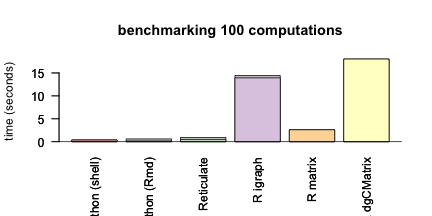``````knitr::opts_chunk\$set(
collapse = TRUE,
comment = "#>",
eval = module
)
``````
``````print(module)
#>  TRUE
``````

# Benchmarking the Leiden Algorithm

In this guide we will run the Leiden algorithm in both R and Python to benchmark performance and demonstrate how the algorithm is called with `reticulate`.

We are testing this in the following environment:

``````paste(Sys.info()[c(4, 2, 1)])
#>  "MacBook-Pro" "19.6.0"      "Darwin"
``````
``````R.version\$version.string
#>  "R version 4.1.0 (2021-05-18)"
``````

## Clustering with the Leiden Algorithm in R

This package allows calling the Leiden algorithm for clustering on an igraph object from R. See the Python and Java implementations for more details:

https://github.com/CWTSLeiden/networkanalysis

https://github.com/vtraag/leidenalg

It calls the Python functions to run the algorithm and passes all arguments need to them.

## Python implementation

The python version can be installed with pip or conda:

``````pip uninstall -y igraph
``````
``````pip install -U -q leidenalg
``````
``````conda install -c vtraag leidenalg
``````

It is also possible to install the python dependencies with reticulate in R.

``````library("reticulate")
py_install("python-igraph")
py_install("leidenalg")
``````

### Running in Python

We are using the following version of Python:

``````import sys
print(sys.version)
#> 3.8.6 | packaged by conda-forge | (default, Oct  7 2020, 18:49:01)
#> [Clang 10.0.1 ]
``````

First we load the packages:

``````import igraph as ig
print("igraph", ig.__version__)
#> igraph 0.8.3
import leidenalg as la
print("leidenalg", la.__version__)
#> leidenalg 0.8.3
``````

Then we load the Zachary karate club example data from igraph.

``````G = ig.Graph.Famous('Zachary')
G.summary()
#> 'IGRAPH U--- 34 78 -- '
``````
``````partition = la.find_partition(G, la.ModularityVertexPartition)
print(partition)
#> Clustering with 34 elements and 4 clusters
#>  8, 9, 14, 15, 18, 20, 22, 26, 29, 30, 32, 33
#>  0, 1, 2, 3, 7, 11, 12, 13, 17, 19, 21
#>  23, 24, 25, 27, 28, 31
#>  4, 5, 6, 10, 16
partition
#> <leidenalg.VertexPartition.ModularityVertexPartition object at 0x7ff48c5bd640>
``````
``````partition.membership
#> [1, 1, 1, 1, 3, 3, 3, 1, 0, 0, 3, 1, 1, 1, 0, 0, 3, 1, 0, 1, 0, 1, 0, 2, 2, 2, 0, 2, 2, 0, 0, 2, 0, 0]
``````
``````partition <- py\$partition\$membership + 1
table(partition)
#> partition
#>  1  2  3  4
#> 12 11  6  5
``````

We can plot the result in R to show it in the network. This reproduces the example in the Python leidenalg documentation.

``````library("igraph")
#>
#> Attaching package: 'igraph'
#> The following objects are masked from 'package:stats':
#>
#>     decompose, spectrum
#> The following object is masked from 'package:base':
#>
#>     union
library("reticulate")
library("RColorBrewer")
graph_object <- graph.famous("Zachary")
node.cols <- brewer.pal(max(c(3, partition)),"Pastel1")[partition]
plot(graph_object, vertex.color = node.cols, layout=layout_with_kk)
``````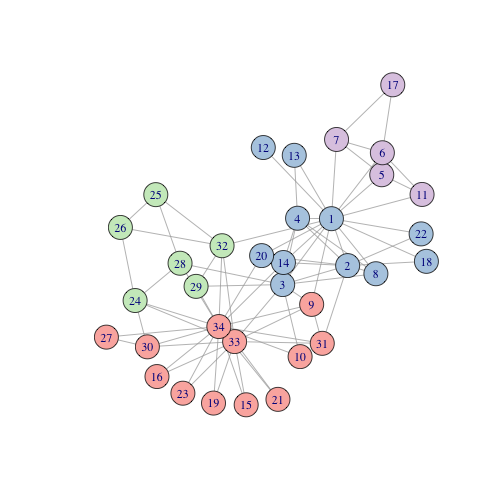We can reproduce passing arguments in this manner as well.

``````partition = la.find_partition(G, la.CPMVertexPartition, resolution_parameter = 0.05)
print(partition)
#> Clustering with 34 elements and 2 clusters
#>  8, 14, 15, 18, 20, 22, 23, 24, 25, 26, 27, 28, 29, 30, 31, 32, 33
#>  0, 1, 2, 3, 4, 5, 6, 7, 9, 10, 11, 12, 13, 16, 17, 19, 21
partition
#> <leidenalg.VertexPartition.CPMVertexPartition object at 0x7ff48c5bddf0>
``````
``````partition.membership
#> [1, 1, 1, 1, 1, 1, 1, 1, 0, 1, 1, 1, 1, 1, 0, 0, 1, 1, 0, 1, 0, 1, 0, 0, 0, 0, 0, 0, 0, 0, 0, 0, 0, 0]
``````
``````partition <- py\$partition\$membership + 1
table(partition)
#> partition
#>  1  2
#> 17 17
``````

We can plot the result in R to show it in the network. This reproduces the example in the Python leidenalg documentation.

``````graph_object <- graph.famous("Zachary")
node.cols <- brewer.pal(max(c(3, partition)),"Pastel1")[partition]
plot(graph_object, vertex.color = node.cols, layout=layout_with_kk)
``````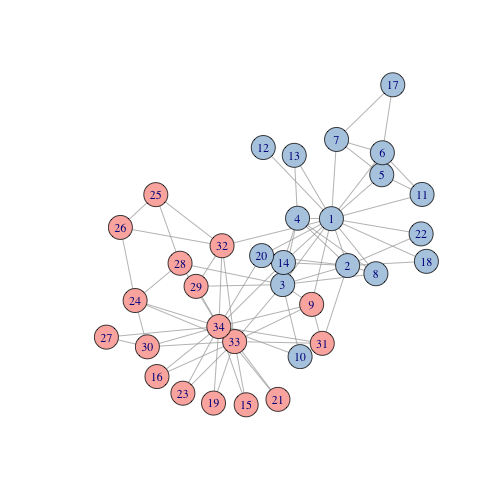We can run the RBC vertex method which generalises the modularity vertex partition.

``````partition = la.find_partition(G, la.RBConfigurationVertexPartition, resolution_parameter = 1.5)
print(partition)
#> Clustering with 34 elements and 5 clusters
#>  8, 14, 15, 18, 20, 22, 26, 29, 30, 32, 33
#>  0, 1, 3, 7, 11, 12, 13, 17, 19, 21
#>  23, 24, 25, 27, 31
#>  4, 5, 6, 10, 16
#>  2, 9, 28
partition
#> <leidenalg.VertexPartition.RBConfigurationVertexPartition object at 0x7ff48c5c3520>
``````
``````partition.membership
#> [1, 1, 4, 1, 3, 3, 3, 1, 0, 4, 3, 1, 1, 1, 0, 0, 3, 1, 0, 1, 0, 1, 0, 2, 2, 2, 0, 2, 4, 0, 0, 2, 0, 0]
``````
``````partition <- py\$partition\$membership + 1
table(partition)
#> partition
#>  1  2  3  4  5
#> 11 10  5  5  3
``````

We can plot the result in R to show it in the network.

``````graph_object <- graph.famous("Zachary")
node.cols <- brewer.pal(max(c(3, partition)),"Pastel1")[partition]
plot(graph_object, vertex.color = node.cols, layout=layout_with_kk)
``````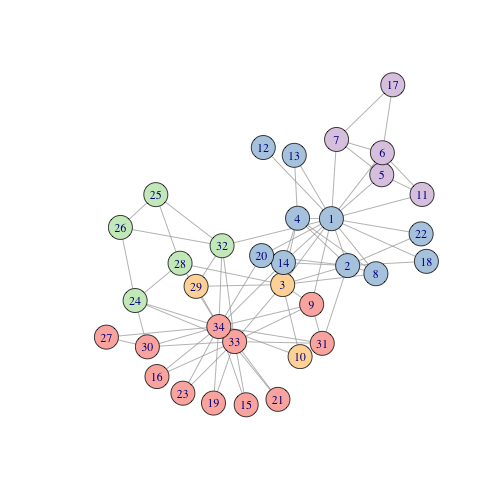#### Benchmarking the Python version with reticulate

Now we can time how long the computation of the algorithm takes (for 1000 runs) running within python:

``````import time
G = ig.Graph.Famous('Zachary')
G.summary()
#> 'IGRAPH U--- 34 78 -- '
start = time.time()
for ii in range(100):
partition = la.find_partition(G, la.ModularityVertexPartition)

end = time.time()
partition.membership
#> [1, 1, 1, 1, 3, 3, 3, 1, 0, 0, 3, 1, 1, 1, 0, 0, 3, 1, 0, 1, 0, 1, 0, 2, 2, 2, 0, 2, 2, 0, 0, 2, 0, 0]
py_time = end - start
print("leiden time:", py_time, "seconds")
#> leiden time: 0.16594791412353516 seconds
``````
``````bash_py_time=`python -c 'import igraph as ig
import leidenalg as la
import time
G = ig.Graph.Famous("Zachary")
G.summary()
start = time.time()
for ii in range(100):
partition = la.find_partition(G, la.ModularityVertexPartition)

end = time.time()
partition.membership
py_time = end - start
print(py_time)'`
echo \$bash_py_time > bash_py_time
echo "leiden time:" \$bash_py_time "seconds"
#> leiden time: 0.39020395278930664 seconds
``````
``````bash_py_time <- as.numeric(readLines("bash_py_time"))
``````

We can also run the leiden algorithm in python by calling functions with reticulate:

``````leidenalg <- import("leidenalg", delay_load = TRUE)
ig <- import("igraph", delay_load = TRUE)
G = ig\$Graph\$Famous('Zachary')
G\$summary()
#>  "IGRAPH U--- 34 78 -- "
partition = leidenalg\$find_partition(G, leidenalg\$ModularityVertexPartition)
partition\$membership
#>   1 1 1 1 3 3 3 1 0 0 3 1 1 1 0 0 3 1 0 1 0 1 0 2 2 2 0 2 2 0 0 2 0 0
``````
``````leidenalg <- import("leidenalg", delay_load = TRUE)
ig <- import("igraph", delay_load = TRUE)
G = ig\$Graph\$Famous('Zachary')
G\$summary()
#>  "IGRAPH U--- 34 78 -- "
start <- Sys.time()
for(ii in 1:100){
partition = leidenalg\$find_partition(G, leidenalg\$ModularityVertexPartition)
}
end <- Sys.time()
partition\$membership
#>   1 1 1 1 3 3 3 1 0 0 3 1 1 1 0 0 3 1 0 1 0 1 0 2 2 2 0 2 2 0 0 2 0 0
reticulate_time <- difftime(end, start)[]
print(paste(c("leiden time:", reticulate_time, "seconds"), collapse = " "))
#>  "leiden time: 0.475473165512085 seconds"
``````

## R implementation

The R version can be installed with devtools or from CRAN:

``````install.packages("leiden")
``````
``````install.packages("leiden")
``````

Note that these require the Python version as a dependency.

### Running in R

We can reproduce these by running the Leiden algorithm in R using the functions in the leiden package.

We are using the following version of R:

``````R.version.string
#>  "R version 4.1.0 (2021-05-18)"
``````

First we load the packages:

``````library("igraph")
library("leiden")
``````

Then we load the Zachary karate club example data from igraph.

``````G <- graph.famous("Zachary")
summary(G)
#> IGRAPH 0a6e55d U--- 34 78 -- Zachary
#> + attr: name (g/c)
``````
``````partition <- leiden(G, "ModularityVertexPartition")
partition
#>   2 2 2 2 3 3 3 2 1 2 3 2 2 2 1 1 3 2 1 2 1 2 1 1 4 4 1 4 4 1 1 4 1 1
``````
``````table(partition)
#> partition
#>  1  2  3  4
#> 12 12  5  5
``````

We can plot the result in R to show it in the network. This reproduces the example in the Python leidenalg documentation.

``````library("igraph")
library("reticulate")
library("RColorBrewer")
node.cols <- brewer.pal(max(c(3, partition)),"Pastel1")[partition]
plot(G, vertex.color = node.cols, layout=layout_with_kk)
``````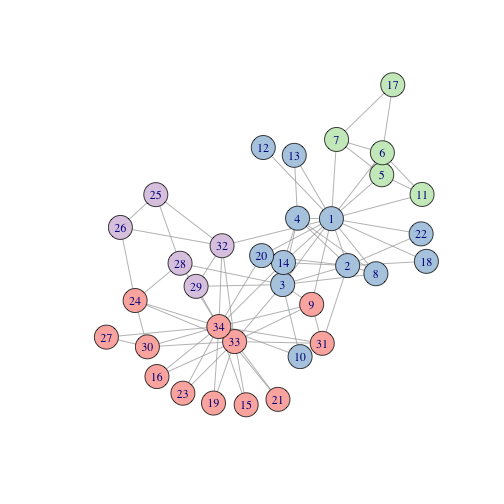We can reproduce passing arguments in this manner as well.

``````partition <- leiden(G, "CPMVertexPartition", resolution_parameter = 0.5)
partition
#>    1  1  1  1  6  3  3  1  2  9  6 10  8  1 11 18  3 12 13 14  2 15 16  5  4
#>   4  7  5 17  7  2  4  2  2
``````
``````table(partition)
#> partition
#>  1  2  3  4  5  6  7  8  9 10 11 12 13 14 15 16 17 18
#>  6  5  3  3  2  2  2  1  1  1  1  1  1  1  1  1  1  1
``````

We can plot the result in R to show it in the network. This reproduces the example in the Python leidenalg documentation.

``````node.cols <- brewer.pal(max(c(3, partition)),"Pastel1")[partition]
#> Warning in brewer.pal(max(c(3, partition)), "Pastel1"): n too large, allowed maximum for palette Pastel1 is 9
#> Returning the palette you asked for with that many colors
plot(G, vertex.color = node.cols, layout=layout_with_kk)
``````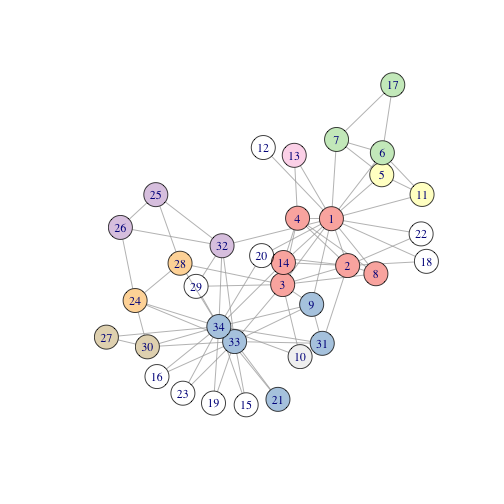We can run the RBC vertex method which generalises the modularity vertex partition.

``````partition <- leiden(G, "RBConfigurationVertexPartition", resolution_parameter = 1.5)
partition
#>   2 2 2 2 4 4 4 2 1 5 4 2 2 2 1 1 4 2 1 2 1 2 1 3 3 3 1 3 3 1 1 3 1 1
``````
``````table(partition)
#> partition
#>  1  2  3  4  5
#> 11 11  6  5  1
``````

We can plot the result in R to show it in the network.

``````node.cols <- brewer.pal(max(c(3, partition)),"Pastel1")[partition]
plot(G, vertex.color = node.cols, layout=layout_with_kk)
``````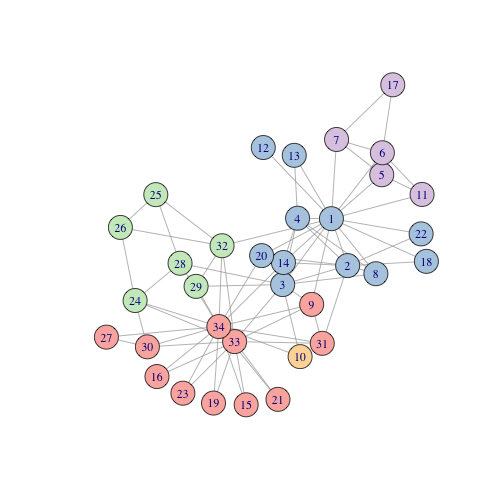#### Benchmarking the R version with reticulate

Now we can time how long the computation of the algorithm takes (for 1000 runs) calling with R on a graph object:

``````G <- graph.famous('Zachary')
summary(G)
#> IGRAPH 60bff49 U--- 34 78 -- Zachary
#> + attr: name (g/c)
start <- Sys.time()
for(ii in 1:100){
partition = leiden(G, "ModularityVertexPartition")
}
end <- Sys.time()
table(partition)
#> partition
#>  1  2  3  4
#> 12 11  6  5
R_graph_time = difftime(end, start)[]
print(paste(c("leiden time:", R_graph_time, "seconds"), collapse = " "))
#>  "leiden time: 13.9325280189514 seconds"
``````

We can see that the R implementation does not perform as well as the Python version but it is convenient for R users. Calling from a graph object avoids casting to a dense adjacency matrix which reduces memory load for large graph objects.

We can see that calling leiden in R on an adjacency matrix has faster performance but it does require more memory. For example, on a dense adjacency matrix:

``````G <- graph.famous('Zachary')
summary(G)
#> IGRAPH b339a63 U--- 34 78 -- Zachary
#> + attr: name (g/c)

start <- Sys.time()
for(ii in 1:100){
adj_mat <- as_adjacency_matrix(G, sparse = FALSE)
}
end <- Sys.time()
dim(adj_mat)
#>  34 34
R_mat_cast_time = difftime(end, start)[]
paste(print(c("cast time:", R_mat_cast_time, "seconds"), collapse = " "))
#>  "cast time:"         "0.0532660484313965" "seconds"
#>  "cast time:"         "0.0532660484313965" "seconds"

start <- Sys.time()
for(ii in 1:100){
partition <- leiden(adj_mat, "ModularityVertexPartition")
}
end <- Sys.time()
table(partition)
#> partition
#>  1  2  3  4
#> 12 11  6  5
R_mat_time = difftime(end, start)[]
print(paste(c("leiden time:", R_mat_time, "seconds"), collapse = " "))
#>  "leiden time: 2.61312413215637 seconds"
``````

For example, on a sparse dgCMatrix for the adjacency matrix:

``````G <- graph.famous('Zachary')
summary(G)
#> IGRAPH 7541afa U--- 34 78 -- Zachary
#> + attr: name (g/c)

start <- Sys.time()
for(ii in 1:100){
adj_mat <- as_adjacency_matrix(G, sparse = TRUE)
}
end <- Sys.time()
class(adj_mat)
#>  "dgCMatrix"
#> attr(,"package")
#>  "Matrix"
dim(adj_mat)
#>  34 34
R_sparse_mat_cast_time = difftime(end, start)[]
paste(print(c("cast time:", R_sparse_mat_cast_time, "seconds"), collapse = " "))
#>  "cast time:"        "0.250636100769043" "seconds"
#>  "cast time:"        "0.250636100769043" "seconds"

start <- Sys.time()
for(ii in 1:100){
partition <- leiden(adj_mat, "ModularityVertexPartition")
}
end <- Sys.time()
table(partition)
#> partition
#>  1  2  3  4
#> 12 11  6  5
R_sparse_mat_time = difftime(end, start)[]
print(paste(c("leiden time:", R_mat_time, "seconds"), collapse = " "))
#>  "leiden time: 2.61312413215637 seconds"
``````

#### Large adjacency matrices

The difference between sparse and dense matrices is more pronounced for large matrices (with few edges):

``````adjacency_matrix <- rbind(cbind(matrix(round(rbinom(1000000, 1, 0.008)), 1000, 1000),
matrix(round(rbinom(1000000, 1, 0.003)), 1000, 1000),
matrix(round(rbinom(1000000, 1, 0.001)), 1000, 1000)),
cbind(matrix(round(rbinom(1000000, 1, 0.003)), 1000, 1000),
matrix(round(rbinom(1000000, 1, 0.008)), 1000, 1000),
matrix(round(rbinom(0000000, 1, 0.002)), 1000, 1000)),
cbind(matrix(round(rbinom(1000000, 1, 0.003)), 1000, 1000),
matrix(round(rbinom(1000000, 1, 0.001)), 1000, 1000),
matrix(round(rbinom(1000000, 1, 0.009)), 1000, 1000)))
rownames(adjacency_matrix) <- 1:3000
colnames(adjacency_matrix) <- 1:3000
G <- graph_from_adjacency_matrix(adjacency_matrix)

start <- Sys.time()
for(ii in 1:10){
adj_mat <- as_adjacency_matrix(G, sparse = FALSE)
}
end <- Sys.time()
class(adj_mat)
#>  "matrix" "array"
dim(adj_mat)
#>  3000 3000
R_mat_large_cast_time = difftime(end, start)[]
paste(print(c("cast time:", R_mat_large_cast_time, "seconds"), collapse = " "))
#>  "cast time:"       "1.40431690216064" "seconds"
#>  "cast time:"       "1.40431690216064" "seconds"

start <- Sys.time()
for(ii in 1:10){
partition <- leiden(adj_mat, "ModularityVertexPartition")
}
end <- Sys.time()
table(partition)
#> partition
#>    1    2    3
#> 1075 1016  909
R_mat_large_time = difftime(end, start)[]
print(paste(c("leiden time:", R_mat_large_time, "seconds"), collapse = " "))
#>  "leiden time: 1.59283774693807 seconds"
``````

For example, on a sparse adjacency matrix:

``````start <- Sys.time()
for(ii in 1:100){
adj_mat <- as_adjacency_matrix(G, sparse = TRUE)
}
end <- Sys.time()
class(adj_mat)
#>  "dgCMatrix"
#> attr(,"package")
#>  "Matrix"
dim(adj_mat)
#>  3000 3000
R_mat_large_cast_time = difftime(end, start)[]
paste(print(c("cast time:", R_mat_large_cast_time, "seconds"), collapse = " "))
#>  "cast time:"       "1.65250110626221" "seconds"
#>  "cast time:"       "1.65250110626221" "seconds"

start <- Sys.time()
for(ii in 1:10){
partition <- leiden(adj_mat, "ModularityVertexPartition")
}
end <- Sys.time()
table(partition)
#> partition
#>    1    2    3
#> 1093 1015  892
R_mat_large_time = difftime(end, start)[]
print(paste(c("leiden time:", R_mat_large_time, "seconds"), collapse = " "))
#>  "leiden time: 5.55539893309275 seconds"
``````

### Comparing the adjacency matrix calling

We compare the processing of adjaceny matrices in the leiden.matrix method to casting to graph in python with reticulate. The current implementation of the R function works as follows. The adjacency matrix is passed to python and the graph object is create in the python-igraph:

``````partition_type <- "RBConfigurationVertexPartition"
initial_membership <- NULL
weights <- NULL
node_sizes = NULL
resolution_parameter = 1

G <- graph.famous('Zachary')
summary(G)
#> IGRAPH 3a393ab U--- 34 78 -- Zachary
#> + attr: name (g/c)
time1 <- Sys.time()
object <- as.matrix(as_adjacency_matrix(G))
time2 <- Sys.time()
timing = difftime(time2, time1)[]
print(paste(c("cast to adjacent:", timing, "seconds"), collapse = " "))
#>  "cast to adjacent: 0.00738215446472168 seconds"

#run matrix method
leidenalg <- import("leidenalg", delay_load = TRUE)
ig <- import("igraph", delay_load = TRUE)

#convert matrix input (corrects for sparse matrix input)
if(is.matrix(object) || is(adj_mat_sparse, "Matrix")){
adj_mat <- object
} else{
adj_mat <- as.matrix(object)
}

#compute weights if non-binary adjacency matrix given
is_pure_adj <- all(as.logical(adj_mat) == adj_mat)
if (is.null(weights) && !is_pure_adj) {
#assign weights to edges (without dependancy on igraph)
t_mat <- t(adj_mat)
weights <- t_mat[t_mat!=0]
#remove zeroes from rows of matrix and return vector of length edges
}

time3 <- Sys.time()
##convert to python numpy.ndarray, then a list
adj_mat_py <- r_to_py(adj_mat)
adj_mat_py <- adj_mat_py\$tolist()
time4 <- Sys.time()
timing = difftime(time4, time3)[]
print(paste(c("pass to python matrix:", timing, "seconds"), collapse = " "))
#>  "pass to python matrix: 0.00565004348754883 seconds"

#convert graph structure to a Python compatible object
GraphClass <- if (!is.null(weights) && !is_pure_adj){
ig\$Graph\$Weighted_Adjacency
} else {
ig\$Graph\$Adjacency
}
time5 <- Sys.time()
snn_graph <- GraphClass(adj_mat_py)
time6 <- Sys.time()
timing = difftime(time6, time5)[]
reticulate_create_time = difftime(time6, time5)[]
print(paste(c("generate graph in python:", timing, "seconds"), collapse = " "))
#>  "generate graph in python: 0.00449204444885254 seconds"

# test performance for computing matrix to graph in R
# other option is to passing snn_graph to Python

time7 <- Sys.time()
#compute partitions
source("../R/find_partition.R")

partition <- find_partition(snn_graph, partition_type = partition_type,
initial_membership = initial_membership ,
weights = weights,
node_sizes = node_sizes,
resolution_parameter = resolution_parameter
)
time8 <- Sys.time()
timing = difftime(time8, time7)[]
print(paste(c("compute partitions:", timing, "seconds"), collapse = " "))
#>  "compute partitions: 0.0891368389129639 seconds"
timing = difftime(time8, time1)[]
print(paste(c("total:", timing, "seconds"), collapse = " "))
#>  "total: 0.166316032409668 seconds"
partition
#>   2 2 2 2 4 4 4 2 1 1 4 2 2 2 1 1 4 2 1 2 1 2 1 3 3 3 1 3 3 1 1 3 1 1
``````

Is it more efficent to pass to create a graph object in R and pass this to python?

``````partition_type <- "RBConfigurationVertexPartition"
initial_membership <- NULL
weights <- NULL
node_sizes = NULL
resolution_parameter = 1

G <- graph.famous('Zachary')
summary(G)
#> IGRAPH 0af0cb9 U--- 34 78 -- Zachary
#> + attr: name (g/c)
time1 <- Sys.time()
object <- as.matrix(as_adjacency_matrix(G))
time2 <- Sys.time()
timing = difftime(time2, time1)[]
print(paste(c("cast to adjacent:", timing, "seconds"), collapse = " "))
#>  "cast to adjacent: 0.00509190559387207 seconds"

#run matrix method
leidenalg <- import("leidenalg", delay_load = TRUE)
ig <- import("igraph", delay_load = TRUE)

time3 <- Sys.time()
##convert to python numpy.ndarray, then a list
object <- graph_from_adjacency_matrix(adj_mat)
time4 <- Sys.time()
timing = difftime(time4, time3)[]
print(paste(c("generate graph in R:", timing, "seconds"), collapse = " "))
#>  "generate graph in R: 0.00300288200378418 seconds"

#convert graph structure to a Python compatible object
time5 <- Sys.time()
##convert to list for python input
if(!is.named(object)){
vertices <- as.list(as.character(V(object)))
} else {
vertices <- as.list(names(V(object)))
}

edges <- as_edgelist(object)
dim(edges)
#>  156   2
edgelist <- list(rep(NA, nrow(edges)))
for(ii in 1:nrow(edges)){
edgelist[[ii]] <- as.character(edges[ii,])
}

snn_graph <- ig\$Graph()
snn_graph\$add_vertices(r_to_py(vertices))
snn_graph\$add_edges(r_to_py(edgelist))
time6 <- Sys.time()
timing = difftime(time6, time5)[]
print(paste(c("pass to python graph:", timing, "seconds"), collapse = " "))
#>  "pass to python graph: 0.111759185791016 seconds"

# test performance for computing matrix to graph in R
# other option is to passing snn_graph to Python

time7 <- Sys.time()
#compute partitions
partition <- find_partition(snn_graph, partition_type = partition_type,
initial_membership = initial_membership ,
weights = weights,
node_sizes = node_sizes,
resolution_parameter = resolution_parameter
)
time8 <- Sys.time()
timing = difftime(time8, time7)[]
print(paste(c("compute partitions:", timing, "seconds"), collapse = " "))
#>  "compute partitions: 0.0217139720916748 seconds"
timing = difftime(time8, time1)[]
print(paste(c("total:", timing, "seconds"), collapse = " "))
#>  "total: 0.159725904464722 seconds"
partition
#>   2 2 2 2 4 4 4 2 1 1 4 2 2 2 1 1 4 2 1 2 1 2 1 3 3 3 1 3 3 1 1 3 1 1
``````

Another approach is to generate a graph in R and pass it to the leiden.igraph method.

``````partition_type <- "RBConfigurationVertexPartition"
initial_membership <- NULL
weights <- NULL
node_sizes = NULL
resolution_parameter = 1

G <- graph.famous('Zachary')
summary(G)
#> IGRAPH 85e99fc U--- 34 78 -- Zachary
#> + attr: name (g/c)
time1 <- Sys.time()
object <- as.matrix(as_adjacency_matrix(G))
time2 <- Sys.time()
timing = difftime(time2, time1)[]
print(paste(c("cast to adjacent:", timing, "seconds"), collapse = " "))
#>  "cast to adjacent: 0.0067451000213623 seconds"

time3 <- Sys.time()
##convert to python numpy.ndarray, then a list
object <- graph_from_adjacency_matrix(adj_mat)
time4 <- Sys.time()
timing = difftime(time4, time3)[]
R_graph_create_time = difftime(time4, time3)[]
print(paste(c("generate graph in R:", timing, "seconds"), collapse = " "))
#>  "generate graph in R: 0.00505685806274414 seconds"

#convert graph structure to a Python compatible object
time5 <- Sys.time()
##convert to list for python input
snn_graph <- object
time6 <- Sys.time()
timing = difftime(time6, time5)[]
print(paste(c("pass to R graph:", timing, "seconds"), collapse = " "))
#>  "pass to R graph: 0.0073540210723877 seconds"

# test performance for computing matrix to graph in R
# other option is to passing snn_graph to Python

time7 <- Sys.time()
#compute partitions
partition <- leiden(snn_graph, partition_type = partition_type,
initial_membership = initial_membership ,
weights = weights,
node_sizes = node_sizes,
resolution_parameter = resolution_parameter
)
time8 <- Sys.time()
timing = difftime(time8, time7)[]
print(paste(c("compute partitions:", timing, "seconds"), collapse = " "))
#>  "compute partitions: 0.258213043212891 seconds"
timing = difftime(time8, time1)[]
print(paste(c("total:", timing, "seconds"), collapse = " "))
#>  "total: 0.307069063186646 seconds"
partition
#>   2 2 2 2 4 4 4 2 1 1 4 2 2 2 1 1 4 2 1 2 1 2 1 3 3 3 1 3 3 1 1 3 1 1
``````

Here we can see that the current approach to pass adjacency matrices to Python and generate graphs in Python is more efficient for a dense matrix than computing the graph in R. Therefore the leiden.matrix method will not call the leiden.igraph method and they will remain distinct.

## Summary

Here we compare the compute time for the Zachary datasets between each method for computing paritions from the leiden clustering algorithm in R or Python.

``````barplot(c(bash_py_time, py\$py_time, reticulate_time, R_graph_time,
R_mat_time, R_sparse_mat_time),
names = c("Python (shell)", "Python (Rmd)", "Reticulate", "R igraph",
"R matrix","R dgCMatrix"),
col = brewer.pal(9,"Pastel1"), las = 2, srt = 45,
ylab = "time (seconds)", main = "benchmarking 100 computations")
abline(h=0)
``````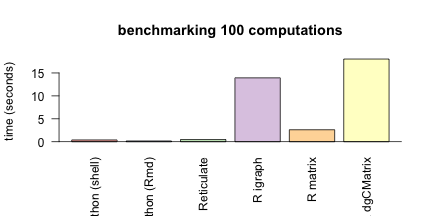If we account for time to cast matrices from graph objects. Then these are the time taken to compute partitions from a graph in R.

``````barplot(c(bash_py_time, py\$py_time, reticulate_time, R_graph_time, R_mat_time+R_mat_cast_time,
R_sparse_mat_time+R_sparse_mat_cast_time),
names = c("Python (shell)", "Python (Rmd)", "Reticulate", "R igraph",
"R matrix","R dgCMatrix"),
col = "grey80", las = 2, srt = 45,
ylab = "time (seconds)", main = "benchmarking 100 computations")
barplot(c(bash_py_time, py\$py_time, reticulate_time, R_graph_time,
R_mat_time,  R_sparse_mat_time),
names = c("Python (shell)", "Python (Rmd)", "Reticulate", "R igraph",
"R matrix","R dgCMatrix"),
col = brewer.pal(9,"Pastel1"), las = 2, srt = 45,
ylab = "time (seconds)", main = "benchmarking 100 computations", add = TRUE)
abline(h=0)
``````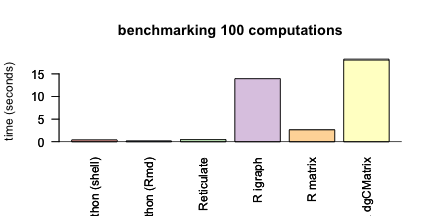Similarly, if we account for time to generate graph from an adjaceny matrix. Then these are the time taken to compute partitions from a matrix in R.

``````R_graph_create_time = difftime(time4, time3)[]
barplot(c(bash_py_time, py\$py_time+reticulate_create_time*100, reticulate_time+reticulate_create_time*100, R_graph_time+R_graph_create_time*100, R_mat_time,
R_sparse_mat_time),
names = c("Python (shell)", "Python (Rmd)", "Reticulate", "R igraph",
"R matrix","R dgCMatrix"),
col = "grey80", las = 2, srt = 45,
ylab = "time (seconds)", main = "benchmarking 100 computations")
barplot(c(bash_py_time, py\$py_time, reticulate_time, R_graph_time,
R_mat_time,  R_sparse_mat_time),
names = c("Python (shell)", "Python (Rmd)", "Reticulate", "R igraph",
"R matrix","R dgCMatrix"),
col = brewer.pal(9,"Pastel1"), las = 2, srt = 45,
ylab = "time (seconds)", main = "benchmarking 100 computations", add = TRUE)
abline(h=0)
``````